#### Approximate value iteration

The continuous-space methods from Section 10.6 can be directly applied to produce an approximate solution by interpolating over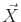to determine cost-to-go values. The initial cost-to-go value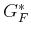over the collection of samples is obtained by (12.6). Following (10.46), the dynamic programming recurrence is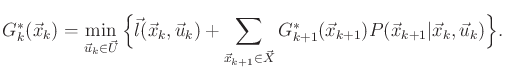(12.10)

If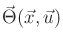is finite, the probability mass is distributed over a finite set of points,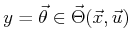. This in turn implies that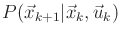is also distributed over a finite subset of. This is somewhat unusual becauseis a continuous space, which ordinarily requires the specification of a probability density function. Since the set of future states is finite, this enables a sum to be used in (12.10) as opposed to an integral over a probability density function. This technically yields a probability density over, but this density must be expressed using Dirac functions.12.1 An approximation is still needed, however, because the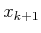points may not be exactly the sample points on which the cost-to-go function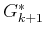is represented.

Steven M LaValle 2020-08-14